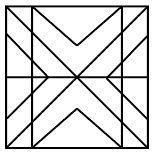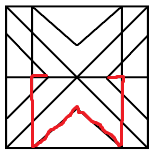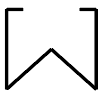Question 6

# Select the option that is embedded in the given figure (rotation is NOT allowed).Solution

Given Figurethen the embedded figure is given belowtherefore figureHence Option (B) Ans